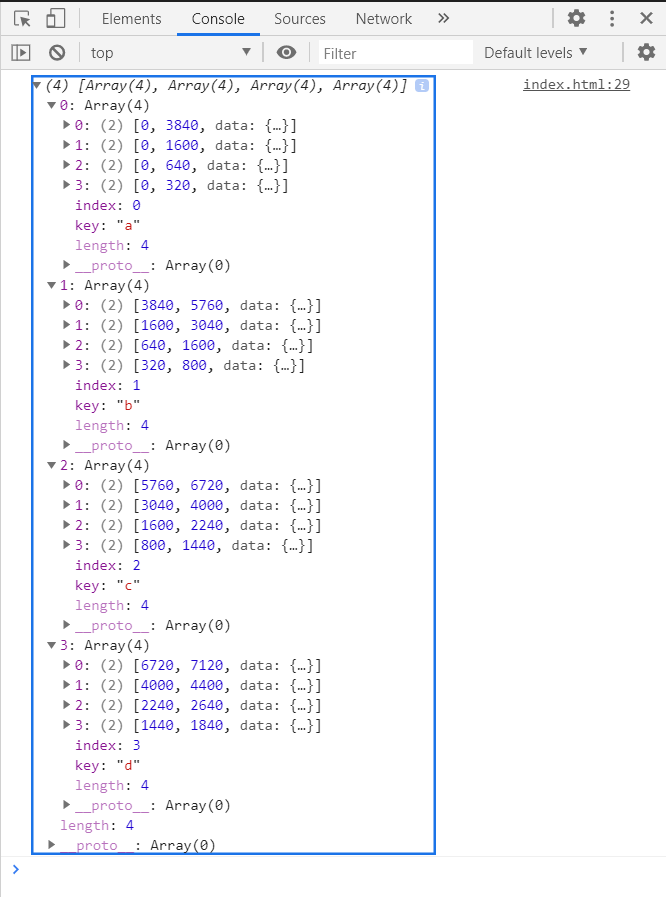Related Articles

# D3.js stackOrderDescending() Method

• Last Updated : 14 Sep, 2020

The d3.stackOrderDescending() method orders the series based on the ordering of the keys as defined by the stack.keys() method. The series with the largest sum with be placed on the bottom, ascending upwards to the smallest.

Syntax:

```d3.stackOrderDescending(series)
```

Parameters: This function accepts a single parameter as mentioned above and described below:

• series: This is the series based on the ordering of the keys to be ordered.

Return Value: This method returns no value.

Example: Ordering the stack using d3.stackOrderDescending function.

## HTML

 ```<``html``>``<``head``>``    ``<``meta` `charset``=``"utf-8"``>` `    ``<``script` `src``=``        ``"https://d3js.org/d3.v5.min.js"``>``    ````` `<``body``>``    ``<``h1` `style``=``"text-align: center; color: green;"``>``        ``GeeksforGeeks``    ``` `    ``<``script``>``        ``var data = [``          ``{a: 3840, b: 1920, c: 960, d: 400},``          ``{a: 1600, b: 1440, c: 960, d: 400},``          ``{a:  640, b:  960, c: 640, d: 400},``          ``{a:  320, b:  480, c: 640, d: 400}``        ``];``        ``var stack = d3.stack()``            ``.keys(["a", "b", "c", "d"])``            ``.order(d3.stackOrderAscending)``            ``.offset(d3.stackOffsetNone);` `        ``var series = stack(data);``        ``console.log(series);` `    ````` ``

Output:My Personal Notes arrow_drop_up